# Hoover Index

## Definition

For the purpose of measuring name, sector or geographic concentration, the Hoover Index is a simple index defined in terms of the absolute deviation from the mean (The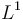$L^1$ norm).

## Details

More precisely, if we have n exposures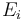$E_i$ summing up to a total exposure of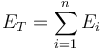$E_T = \sum^{n}_{i=1} E_{i}$

an average exposure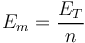$E_m = \frac{E_T}{n}$

and fractional exposures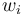$w_i$ are defined as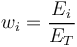$w_{i} = \frac{E_i}{E_T}$

Then the Hoover index is defined as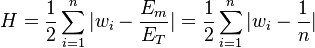$H = \frac{1}{2} \sum^{n}_{i=1} | w_i - \frac{E_m}{E_T} | = \frac{1}{2} \sum^{n}_{i=1} | w_i - \frac{1}{n} |$

None

None

None

## Implementations

Open Source implementations of the Hoover index are available in